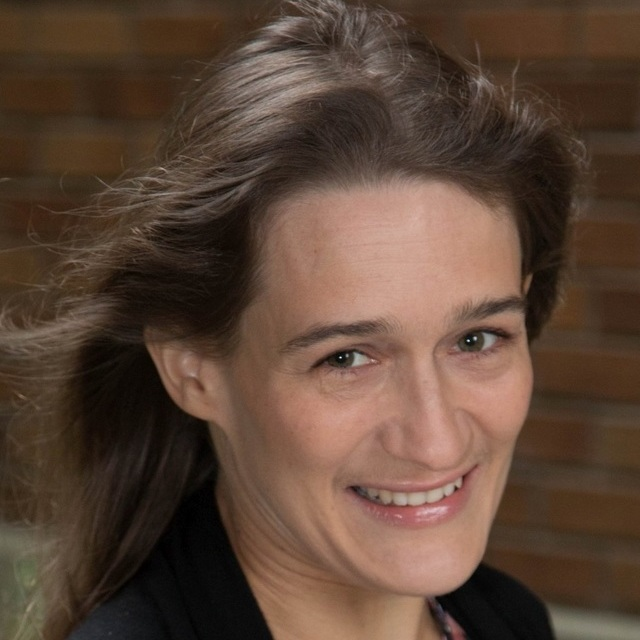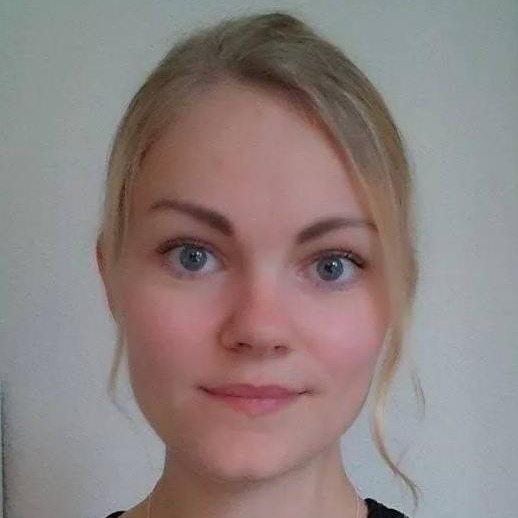This event has ended. Visit the official site or create your own event on Sched.
Wednesday, June 29 • 1:00pm - 1:18pm

Non-linear mixed models of various kinds are fundamental extensions of the linear mixed models commonly used in a wide range of applications. An important example of a non-linear mixed model is the so-called multiplicative mixed model, which we will consider as a model with a linear mixed model part and one or more multiplicative terms. A multiplicative term is here a product of a random effect and a fixed effect, i.e. a term that models a part of the interaction as a random coefficient model based on linear regression on the fixed effect. The multiplicative mixed model can be applied in many different fields for improved statistical inference, e.g. sensory and consumer data, genotype-by-environment data, and data from method comparison studies in medicine. However, the maximum likelihood estimation of the model parameters can be time consuming without proper estimation methods. Using automatic differentiation techniques, the Template Model Builder (TMB) R-package [Kristensen, 2014] fits mixed models through user-specified C++ templates in a very fast manner, making it possible to fit complex models with up to \$10^6\$ random effects within reasonable time. The mumm R-package uses the TMB package to fit multiplicative mixed models, such that the user avoids the coding of C++ templates. The package provides a function, where the user only has to give a model formula and a data set as input to get the multiplicative model fit together with standard model summaries such as parameter estimates and standard errors as output.

ModeratorsPatrícia Martinková

Researcher, Institute of Computer Science, Czech Academy of Sciences
Researcher in statistics and psychometrics from Prague. Uses R to boost active learning in classes. Fulbright alumna and 2013-2015 visiting research scholar with Center for Statistics and the Social Sciences and Department of Statistics, University of Washington.

SpeakersSofie Pødenphant Jensen

PhD Student, Technical University of Denmark